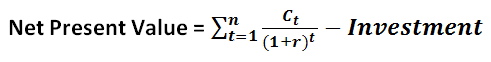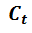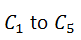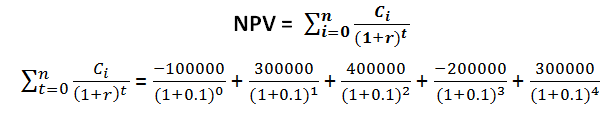# Net Present Value

Present Value implies the present value of a sum of money receivable at a future time. The money receivable in the future may be a cash flow payable over a period or a one-time return.

• Net Present Value is the difference between the initial amount of an investment and the present value of all future tax-free cash flows.
• The value of money changes with time, an amount of money today may value differently after a few months or years, hence while evaluating the projects based on their return it is necessary to bring all payments at one point of time and then calculate the return.

#### Illustration 1:

Ram has taken a loan of Rs. 10000 from the bank at a rate of 10% per year, he invested it in a project that gives him Rs. 10500 at the end of 1 year. Calculate the profitability of the project.
Solution:
Amount Invested = Rs 10000.00; Return Expected = Rs 10500.00
Rate of Return = 500/10000 = 0.05 or 5%
At first look, it seems to be a profitable venture offering a 5% return in 1 year, but when we analyze further,
Future Value of Amount Invested = 110% of Rs. 10000 = Rs 11000.
Hence, Revised Profit = - 500 or 5% loss.
From the illustration, it can be concluded that in order to calculate actual return we either need to find the future value of the investment or the present value of the returns expected.

## Formula

For a project with one-time investment, and returns distributed over a period in the form of cash flows, the Present Value of all Future Cash Flows Receivable is:Where,is the cash flow adjusted with tax at time t.
r is the expected rate of return on the investment
n is the total number of years for which project will generate outflows
Investment is the total one-time amount required for the project at the beginning.
If NPV > 0, then the Project/Investment is profitable
And If NPV < 0, then the Project/Investment is a loss-making venture.
Example 1: ABC Ltd. has received a proposal for investment wherein, the investment of Rs. 10 Lacs is payable fully at the beginning and the project is expected to generate tax-free cash flows as following, the rate of return in the market is 5%. Calculate the viability of the project.
 Year 1 Year 2 Year 3 Year 4 Year 5 220000.00 230000.00 240000.00 200000.00 200000.00
Solution:
Investment = Rs. 1000000.00; r = 0.05, n = 5 years,are given in the above table. Substituting the values in formula
= 209523.81 + 208616.78 + 207321.02 + 164540.49 + 156705.23 - 1000000
Hence, NPV = -53292.67
As NPV < 0, this project is a loss-making venture.
• Sometimes it might be the situation where the company makes investment in parts over a period and the returns are also generated over the period. In such cases, the NPV calculation is slightly different as we need to consider the Present Values of both Investments as well as Return.
Example 2: XYZ Ltd. receives an investment proposal of three years with the following investment and return pattern, if the rate of return is 10%, calculate the viability of the project.
 Year 0 Year 1 Year 2 Year 3 Year 4 Cash Flow -100000.00 300000.00 400000.00 -200000.00 300000.00
Solution:
In above cases where, there are multiple cash inflows and cash outflows, the formula becomes= -100000 + 272727.27 + 330578.51 – 150262.96 + 204904.04
= 557946.86
NPV = 557946.86
As NPV > 0, the project is a profitable venture.